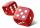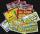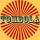Event probability

The probability of event P in 8 independent experiments is 0.33. What is the probability that the event P occurs in one experiment (probability is same)?

Result

p =  87.1 %

Solution:Leave us a comment of example and its solution (i.e. if it is still somewhat unclear...):Be the first to comment!To solve this example are needed these knowledge from mathematics:

Our percentage calculator will help you quickly calculate various typical tasks with percentages. Would you like to compute count of combinations?

Next similar examples:

1. The diceWhat is the probability of events that if we throw a dice is rolled less than 6?
2. Sum 10What is the probability that two dice throw twice in a row will result the sum of 10?
3. Probability - ticketsWhat is the probability that when you have 25 tickets in 5000 that you wins the first (one) prize?
4. Win in raffleThe raffle tickets were sold 200, 5 of which were winning. What is the probability that Peter, who bought one ticket will win?
5. AceFrom complete sets of playing cards (32 cards) we pulled out one card. What is the probability of pulling the ace?
6. Theorem proveWe want to prove the sentence: If the natural number n is divisible by six, then n is divisible by three. From what assumption we started?
7. Percentages52 is what percent of 93?
8. PersonsPersons surveyed:100 with result: Volleyball=15% Baseball=9% Sepak Takraw=8% Pingpong=8% Basketball=60% Find the average how many like Basketball and Volleyball. Please show your solution.Calculate how many percent will increase the length of an HTML document, if any ASCII character unnecessarily encoded as hexadecimal HTML entity composed of six characters (ampersand, grid #, x, two hex digits and the semicolon). Ie. space as: &#x20;
10. Profit gainIf 5% more is gained by selling an article for Rs. 350 than by selling it for Rs. 340, the cost of the article is:
11. CalculationHow much is sum of square root of six and the square root of 225?
12. The ballThe ball was discounted by 10 percent and then again by 30 percent. How many percent of the original price is now?
13. PowersExpress the expression ? as the n-th power of the base 10.
14. Highway repairThe highway repair was planned for 15 days. However, it was reduced by 30%. How many days did the repair of the highway last?
15. PowerNumber ?. Find the value of x.
16. One halfOne half of ? is: ?
17. PercentsHow many percents is 900 greater than the number 750?